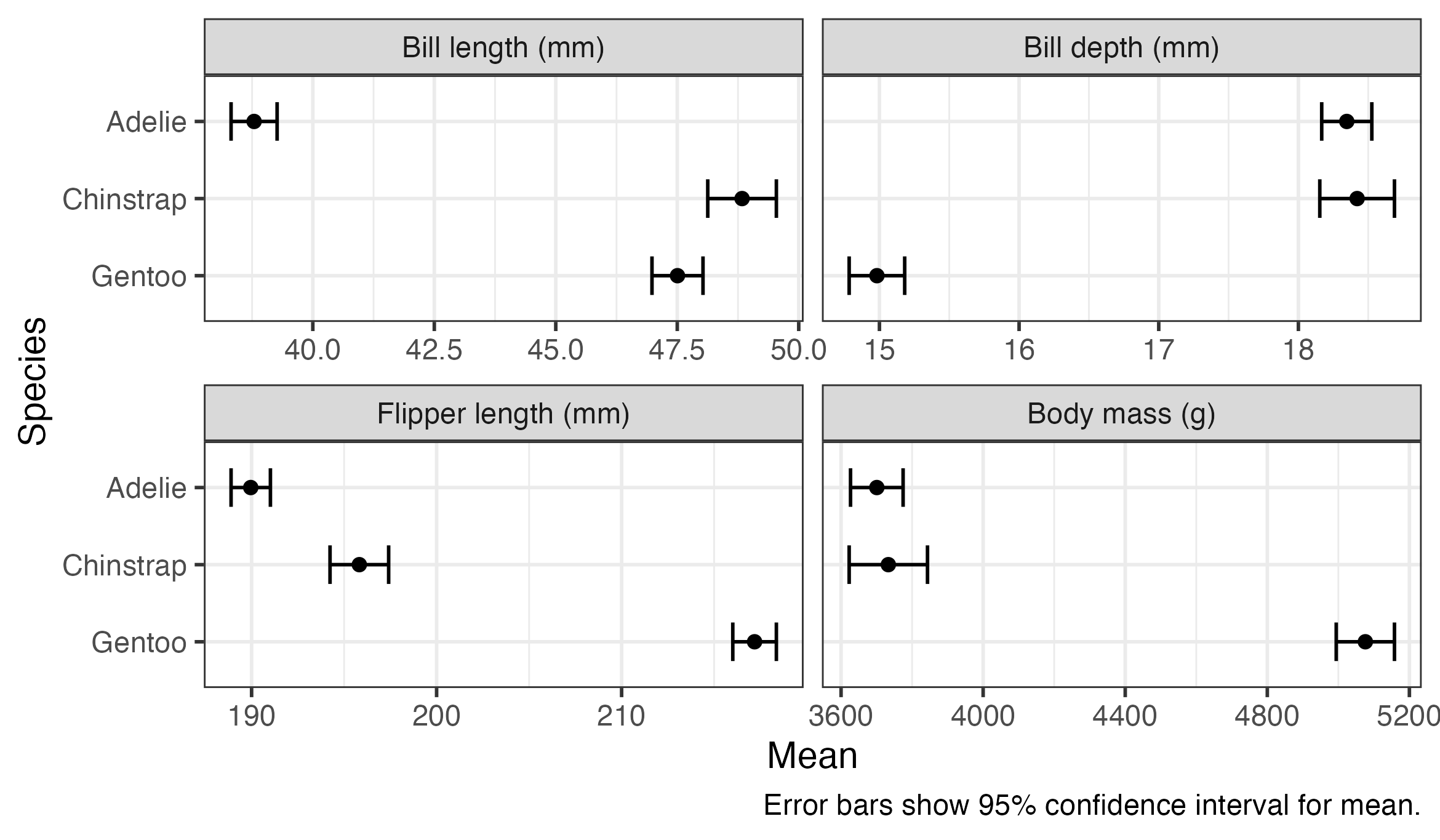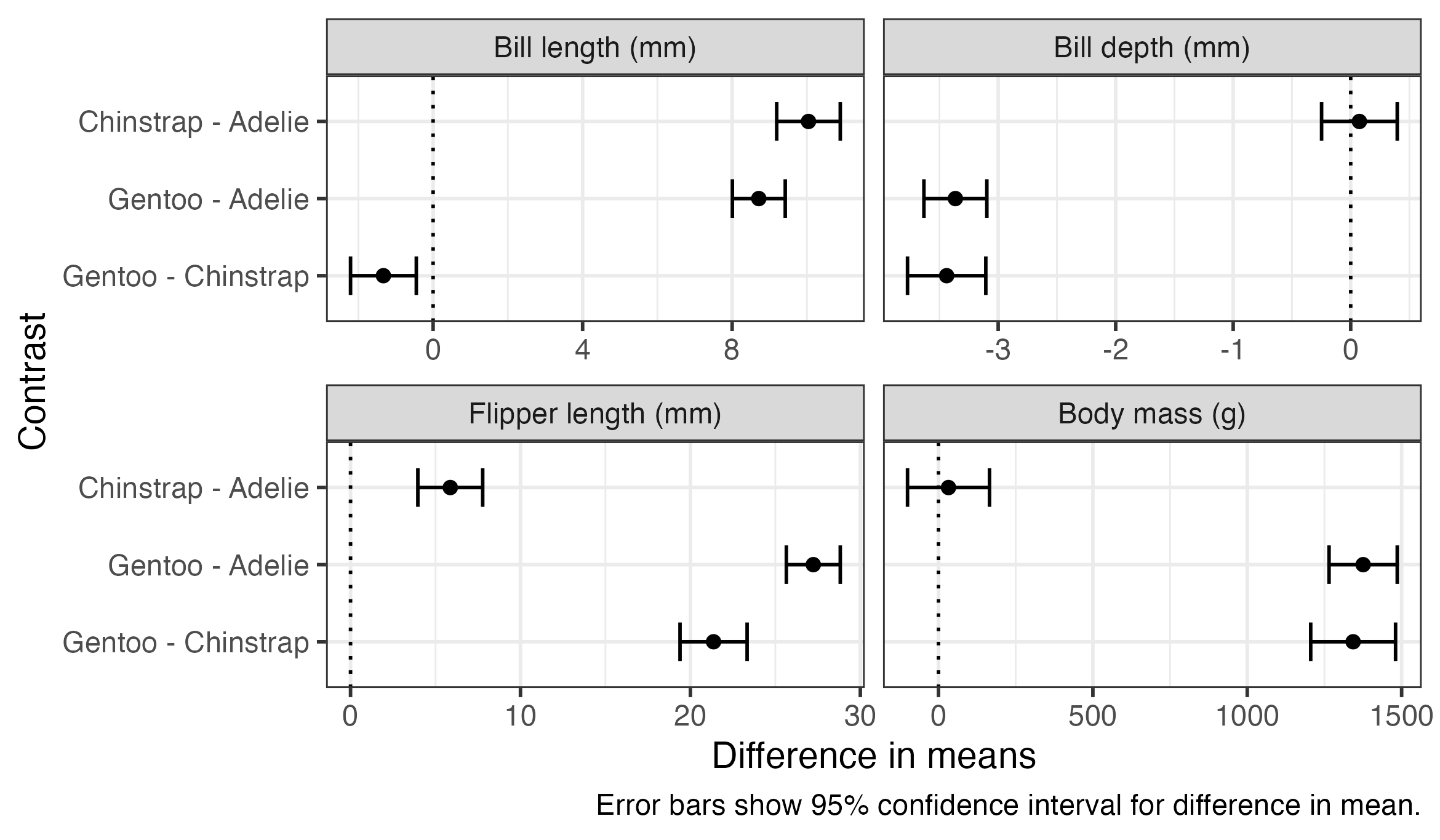# Fitting many statistical models at once using dplyr

r
statistical-models
Author

Cameron Patrick

Published

8 June, 2023

One common task in applied statistics is to fit and interpret a number of statistical models at once. For example, fitting a model with the same structure to a number of different outcome or explanatory variables, or fitting several models with different structure to the same data. Here are some examples of how I usually do this, using features that were introduced with `dplyr` version 1.1.0.

For this demonstration, we’ll be using the R packages `dplyr`, `tidyr`, `ggplot2` (all of which are included in the `tidyverse`), as well as `gt` for making tables, `emmeans` for obtaining estimated means and comparisons, and `performance` for model-checking.

``````library(tidyverse)
library(gt)
library(emmeans)
library(performance)``````

We’ll be using the Palmer Penguins data collected at Palmer Station, Antarctica, made available by Dr Kristen Gorman, and conveniently accessible in R using the `palmerpenguins` package. This dataset contains measurements on a number of penguins of different species on different islands.

``````library(palmerpenguins)
data(penguins)
``````# A tibble: 6 × 8
species island    bill_length_mm bill_depth_mm flipper_length_mm body_mass_g
<fct>   <fct>              <dbl>         <dbl>             <int>       <int>
1 Adelie  Torgersen           39.1          18.7               181        3750
2 Adelie  Torgersen           39.5          17.4               186        3800
3 Adelie  Torgersen           40.3          18                 195        3250
4 Adelie  Torgersen           NA            NA                  NA          NA
5 Adelie  Torgersen           36.7          19.3               193        3450
6 Adelie  Torgersen           39.3          20.6               190        3650
# ℹ 2 more variables: sex <fct>, year <int>``````

Suppose we want to compare bill length, bill depth, flipper length, and body mass between species. We could manually run a separate model for each, but here’s a way to to automate the process. As with many things in R, the trick to doing this easily is to get the data in long form, with the outcomes stacked on top of each other, and a variable indicating which outcome is being measured.

The `pivot_longer()` function from `tidyr` gets the data into this format. I’ve also taken an extra step of recoding the “outcome” variable to give more descriptive labels. This isn’t required but it will make the tables and plots that we make later look nicer.

``````penguins_long <- penguins %>%
pivot_longer(
c(bill_length_mm, bill_depth_mm, flipper_length_mm, body_mass_g),
names_to = "outcome",
values_to = "value"
) %>%
mutate(
outcome = fct_inorder(outcome),
outcome = fct_recode(
outcome,
"Bill length (mm)" = "bill_length_mm",
"Bill depth (mm)" = "bill_depth_mm",
"Flipper length (mm)" = "flipper_length_mm",
"Body mass (g)" = "body_mass_g"
)
)``````

Looking at the first few rows of this data frame, you can see we now have four rows for each penguin, one for each type of measurement:

``head(penguins_long)``
``````# A tibble: 6 × 6
species island    sex     year outcome              value
<fct>   <fct>     <fct>  <int> <fct>                <dbl>
1 Adelie  Torgersen male    2007 Bill length (mm)      39.1
2 Adelie  Torgersen male    2007 Bill depth (mm)       18.7
3 Adelie  Torgersen male    2007 Flipper length (mm)  181
4 Adelie  Torgersen male    2007 Body mass (g)       3750
5 Adelie  Torgersen female  2007 Bill length (mm)      39.5
6 Adelie  Torgersen female  2007 Bill depth (mm)       17.4``````

We can use `group_by()` and `summarise()` from the `dplyr` package to group the rows by outcome, and then “summarise” these groups of rows down to a single row containing a statistical model for each outcome. This makes use of a couple of R tricks: ‘list columns’, a column in a data frame that contains a more complex object than the standard R data types (numeric, character, etc); and the new `pick()` verb which returns a data frame containing selected columns (in this case, all of them) within the group that’s being operated on.

``````penguin_models <- penguins_long %>%
group_by(outcome) %>%
summarise(
model = list(
lm(value ~ species, data = pick(everything()))
)
)``````

You can see the resulting data from contains four rows, one for each outcome, and a statistical model (“lm”) for each:

``print(penguin_models)``
``````# A tibble: 4 × 2
outcome             model
<fct>               <list>
1 Bill length (mm)    <lm>
2 Bill depth (mm)     <lm>
3 Flipper length (mm) <lm>
4 Body mass (g)       <lm>  ``````

Ideally we would also do a visual check of model assumptions. One way to do this is something like the code below, which saves a residual plot for each model to an image file, which can be inspected later. It uses the `check_model()` function in the `performance` package to generate the plots. The generated residual plots aren’t shown here, but they all look fine.

``````walk2(
penguin_models\$outcome,
penguin_models\$model,
~ ggsave(
paste0(.x, ".png"),
plot(check_model(.y, check = c("pp_check", "linearity",
"homogeneity", "qq"))),
width = 12,
height = 9
)
)``````

Once we’ve fitted the models, we can obtain quantities of interest from them. In this example we’ll look at estimated means for each species, p-values testing the hypothesis that all species means are equal (against at least one pair of means being different), and comparisons (differences in means) between all pairs of species.

The `reframe()` function from `dplyr` allows us to run some code that produces a data frame on each model and stack the results on top of each other. We can use the `emmeans()` function from the `emmeans` package to obtain estimated marginal means and `as_tibble()` to convert the result into a data frame. The `rowwise(outcome)` at the start tells `reframe()` that we want to call `emmeans()` separately for each row of the data frame (i.e., each outcome model), and preserve the `outcome` variable in the result.

``````penguin_means <- penguin_models %>%
rowwise(outcome) %>%
reframe(
emmeans(model, "species") %>%
as_tibble()
)``````

The first few rows of the resulting data frame are shown below. There is a row for each outcome for each species, containing the mean (`emmean`), standard error (`SE`), degrees of freedom (`df`) and lower and upper confidence limits (`lower.CL` and `upper.CL`).

``head(penguin_means)``
``````# A tibble: 6 × 7
outcome          species   emmean     SE    df lower.CL upper.CL
<fct>            <fct>      <dbl>  <dbl> <dbl>    <dbl>    <dbl>
1 Bill length (mm) Adelie      38.8 0.241    339     38.3     39.3
2 Bill length (mm) Chinstrap   48.8 0.359    339     48.1     49.5
3 Bill length (mm) Gentoo      47.5 0.267    339     47.0     48.0
4 Bill depth (mm)  Adelie      18.3 0.0912   339     18.2     18.5
5 Bill depth (mm)  Chinstrap   18.4 0.136    339     18.2     18.7
6 Bill depth (mm)  Gentoo      15.0 0.101    339     14.8     15.2``````

We can use `ggplot()` to present the results visually. The plot shows that there’s a substantial variation between species in means of all of these measurements, with little or no overlap between many of the confidence intervals. Gentoo penguins appear to be heavier, and have longer flippers but shorter and shallower bills, than the other species.

``````penguin_means %>%
ggplot(aes(x = emmean, y = species, xmin = lower.CL, xmax = upper.CL)) +
geom_errorbar(width = 0.5) +
geom_point() +
scale_y_discrete(limits = rev) +
facet_wrap(vars(outcome), nrow = 2, scales = "free_x") +
labs(
x = "Mean",
y = "Species",
caption = "Error bars show 95% confidence interval for mean."
)``````The `gt()` function can be used to produce a nice table of results. The code shown below combines the `lower.CL` and `upper.CL` columns to produce a single column with the confidence interval, and separately specifies fewer decimal places for body mass than the other measures. The `group_by()` function before `gt()` results in a table sub-heading for each outcome. You could easily change this to `group_by(species)` to arrange the results by species.

``````penguin_means %>%
group_by(outcome) %>%
gt() %>%
fmt_number(c(emmean, SE, lower.CL, upper.CL),
decimals = 1, use_seps = FALSE) %>%
fmt_number(c(emmean, SE, lower.CL, upper.CL),
rows = outcome == "Body mass (g)",
decimals = 0, use_seps = FALSE) %>%
fmt_number(df, decimals = 0) %>%
cols_align("left", species) %>%
cols_merge_range(lower.CL, upper.CL, sep = ", ") %>%
cols_label(
species = "Species",
emmean = "Mean",
lower.CL = "95% Confidence Interval"
)``````
Species Mean SE df 95% Confidence Interval
Bill length (mm)
Adelie 38.8 0.2 339 38.3, 39.3
Chinstrap 48.8 0.4 339 48.1, 49.5
Gentoo 47.5 0.3 339 47.0, 48.0
Bill depth (mm)
Adelie 18.3 0.1 339 18.2, 18.5
Chinstrap 18.4 0.1 339 18.2, 18.7
Gentoo 15.0 0.1 339 14.8, 15.2
Flipper length (mm)
Adelie 190.0 0.5 339 188.9, 191.0
Chinstrap 195.8 0.8 339 194.2, 197.4
Gentoo 217.2 0.6 339 216.0, 218.4
Body mass (g)
Adelie 3701 38 339 3627, 3775
Chinstrap 3733 56 339 3623, 3843
Gentoo 5076 42 339 4994, 5158

We can do similar to produce an overall “F” test for each model, testing the hypothesis that all species have equal means for a particular measure against the hypothesis that at least one pair of means is different. The `joint_tests()` function in `emmeans` does this.

``````penguin_tests <- penguin_models %>%
rowwise(outcome) %>%
reframe(
joint_tests(model) %>%
as_tibble()
)``````

The resulting data frame is shown below. This time there is one row per model, but if there had been multiple variables in the model, there would have been one row per variable or interaction term (distinguished by the `model term` column). Each row contains the results of a hypothesis test: numerator and denominator degrees of freedom (`df1` and `df2`), F-statistics (`F.ratio`) and p-value (`p.value`).

``print(penguin_tests)``
``````# A tibble: 4 × 6
outcome             `model term`   df1   df2 F.ratio   p.value
<fct>               <chr>        <dbl> <dbl>   <dbl>     <dbl>
1 Bill length (mm)    species          2   339    411. 2.69e- 91
2 Bill depth (mm)     species          2   339    360. 1.51e- 84
3 Flipper length (mm) species          2   339    595. 1.35e-111
4 Body mass (g)       species          2   339    344. 2.89e- 82``````

Again, this can be presented in a table using `gt()`:

``````penguin_tests %>%
gt() %>%
fmt_number(F.ratio, decimals = 1) %>%
fmt_number(p.value, decimals = 3) %>%
cols_merge_range(df1, df2, sep = ", ") %>%
sub_small_vals(p.value, threshold = 0.001) %>%
cols_label(
outcome = "Outcome",
`model term` = "Predictor",
df1 = "df",
F.ratio = "F",
p.value = "p-value"
)``````
Outcome Predictor df F p-value
Bill length (mm) species 2, 339 410.6 <0.001
Bill depth (mm) species 2, 339 359.8 <0.001
Flipper length (mm) species 2, 339 594.8 <0.001
Body mass (g) species 2, 339 343.6 <0.001

Finally, we often want to obtain comparisons between particular estimated quantities. In this example we use the `emmeans` package again for this, this time using the `pairs()` function to produce comparisons between all pairs of species.

``````penguin_pairs <- penguin_models %>%
rowwise(outcome) %>%
reframe(
emmeans(model, "species") %>%
pairs(infer = TRUE, reverse = TRUE, adjust = "none") %>%
as_tibble()
)``````

The first few rows of the data frame are shown below. The contents are similar to what we saw earlier for the estimated means, but this time each row contains information on a difference between pairs of means (described in the `contrast` column), along with the estimated difference in means, standard error, degrees of freedom, confidence interval, t-statistic and p-value.

``head(penguin_pairs)``
``````# A tibble: 6 × 9
outcome       contrast estimate    SE    df lower.CL upper.CL t.ratio  p.value
<fct>         <chr>       <dbl> <dbl> <dbl>    <dbl>    <dbl>   <dbl>    <dbl>
1 Bill length … Chinstr…  10.0    0.432   339    9.19    10.9    23.2   4.23e-72
2 Bill length … Gentoo …   8.71   0.360   339    8.01     9.42   24.2   5.33e-76
3 Bill length … Gentoo …  -1.33   0.447   339   -2.21    -0.449  -2.97  3.18e- 3
4 Bill depth (… Chinstr…   0.0742 0.164   339   -0.248    0.396   0.453 6.50e- 1
5 Bill depth (… Gentoo …  -3.36   0.136   339   -3.63    -3.10  -24.7   7.93e-78
6 Bill depth (… Gentoo …  -3.44   0.169   339   -3.77    -3.11  -20.3   1.59e-60``````

These comparisons can be plotted or presented in a table using code very similar to what we used for the estimated means. The plot below also includes a dotted line indicating zero difference, which can be used as a visual indicator for whether comparisons are statistically significant.

``````penguin_pairs %>%
ggplot(aes(x = estimate, y = contrast, xmin = lower.CL, xmax = upper.CL)) +
geom_vline(xintercept = 0, linetype = "dotted") +
geom_errorbar(width = 0.5) +
geom_point() +
scale_y_discrete(limits = rev) +
facet_wrap(vars(outcome), nrow = 2, scales = "free_x") +
labs(
x = "Difference in means",
y = "Contrast",
caption = "Error bars show 95% confidence interval for difference in mean."
)``````